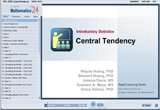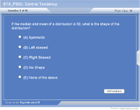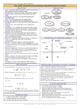How to Learn in 24 Hours?The Rapid Learning Movie

 Need Help? M-F: 9am-5pm(PST): Toll-Free: (877) RAPID-10 US Direct: (714) 692-2900 Int'l: 001-714-692-2900 24/7 Online Technical Support: The Rapid Support Center Secure Online Order:Need Proof? Testimonials by Our Users

 Rapid Learning Courses: MCAT in 24 Hours (2015-16) USMLE in 24 Hours (Boards) Chemistry in 24 Hours Biology in 24 Hours Physics in 24 Hours Mathematics in 24 Hours Psychology in 24 Hours SAT in 24 Hours ACT in 24 Hours AP in 24 Hours CLEP in 24 Hours DAT in 24 Hours (Dental) OAT in 24 Hours (Optometry) PCAT in 24 Hours (Pharmacy) Nursing Entrance Exams Certification in 24 Hours eBook - Survival Kits Audiobooks (MP3)

 Tell-A-Friend: Have friends taking science and math courses too? Tell them about our rapid learning system.Home »  Mathematics »  Introductory Statistics

Central Tendency

 Topic Review on "Title": Median Characteristics: Resistant to outliers Best for skewed distributions Calculating Median To calculate the median with an odd count: Order the observations. Find position of median value with formula, (n + 1) / 2. Determine the value represented by the median position. To calculate the median with an even count: Order the observations. Find position of the middle two values with formulas,   (n / 2) and (n / 2) + 1. Determine values represented by two middle positions. Take average of two middle values to get the median. Mean Characteristics Non-resistant to outliers Best for symmetric distributions Formula: x-bar = Sx/n Calculating Mean Find the sum of all observations in the data set Divide the sum by the count to obtain the mean Calculating Mode Order the observations Report the value in the data set that occurs the most

Rapid Study Kit for "Title":
 Flash Movie Flash Game Flash Card Core Concept Tutorial Problem Solving Drill Review Cheat Sheet"Title" Tutorial Summary : This tutorial describes central tendency and their use in application problems. You will learn how to find the center of a distribution, compute the mean and median of a set of data. You will also understand how outliers affect the median and mean. In addition, we will show you in the tutorial how to determine when to use mean, when to use the median and when to determine the shape of a distribution from the mean and median values.

 Tutorial Features: Specific Tutorial Features: Step by step examples which show what is central tendency, how to calculate median, mean, etc., are included in this tutorial. Examples displaying how to determine the shape are also presented with detailed step by step instructions in this tutorial. Series Features: Concept map showing inter-connections of new concepts in this tutorial and those previously introduced. Definition slides introduce terms as they are needed. Visual representation of concepts Animated examples—worked out step by step A concise summary is given at the conclusion of the tutorial.

 "Title" Topic List: Center: approximately the middle of the distribution, measured with the mean or median. Count: or n, the number of observations in a data set. Median: the middle value of data, with half of the data above it and half below. Mean: the sum of all data points divided by the count. Mode: the observation (number) that occurs most frequently in the data set.

See all 24 lessons in Introductory Statistics, including concept tutorials, problem drills and cheat sheets:
Teach Yourself Introductory Statistics Visually in 24 Hours